GeeksforGeeks App
Open AppBrowser
Continue

# Hibernate – Many-to-Many Mapping

In RDBMS, we can see a very common usage of parent-child relationships. It can be achieved in Hibernate via

1. One-to-many relationship
2. Many-to-one relationship
3. One-to-one relationship
4. Many-to-many relationship

Here we will be discussing how to perform Hibernate – Many-to-Many mappings. Below are the example tables to demonstrate Many-to-Many mappings as listed below:

• geekEmployeeData
• SkillsetData
• geekEmployeeSkill

Implementation:

### A. Steps for  Table creation are as follows:

```-- Table containing employeedata
create table geeksforgeeks.geekEmployeeData (
id INT NOT NULL auto_increment,
firstName VARCHAR(20) default NULL,
lastName  VARCHAR(20) default NULL,
salary     INT  default NULL,
PRIMARY KEY (id)
);```
```-- Table containing skillsets
create table geeksforgeeks.SkillsetData (
id INT NOT NULL auto_increment,
skillName VARCHAR(30) default NULL,
PRIMARY KEY (id)
);```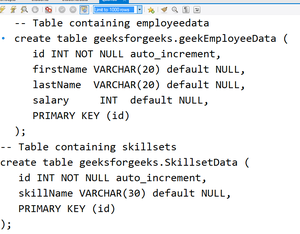```-- Table which can combine employeedata and skillsets
create table geekEmployeeSkill (
geekEmpId INT NOT NULL,
skillId INT NOT NULL,
PRIMARY KEY (geekEmpId,skillId)
);```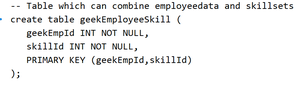geekEmployeeSkill table creation

A Many-to-Many mapping has to be implemented using a Set java collection and it should not contain any duplicate element. A Set can be mapped with a <set> element in the mapping table. It can be initialized with java.util.HashSet.

### B. POJO classes

For geekEmployeeData table data CRUD operations, this is required

Example:

## Java

 `// Java Program to Illustrate GeekEmployeeData Class` `// Importing required classes``import` `java.util.Set;` `// Class``public` `class` `GeekEmployeeData {` `    ``// Attributes are mapping to geekEmployeeData` `    ``// geekEmployeeData.id``    ``private` `int` `id;``  ` `    ``// geekEmployeeData.firstName``    ``private` `String firstName;``  ` `    ``// geekEmployeeData.lastName``    ``private` `String lastName;``  ` `    ``// geekEmployeeData.salary``    ``private` `int` `salary;` `    ``// Collection of skillsets in Set variable``    ``private` `Set skillSets;` `    ``// Method``    ``public` `Set getSkillSets() { ``return` `skillSets; }` `    ``public` `void` `setSkillSets(Set skillSets)``    ``{` `        ``// This keyword refers to current instance itself``        ``this``.skillSets = skillSets;``    ``}` `    ``// Constructor 1``    ``public` `GeekEmployeeData() {}` `    ``// Constructor 2``    ``public` `GeekEmployeeData(String firstName,``                            ``String lastName, ``int` `salary)``    ``{` `        ``// This keyword refers to current instance itself``        ``this``.firstName = firstName;``        ``this``.lastName = lastName;``        ``this``.salary = salary;``    ``}` `    ``// Getter  and Setters` `    ``public` `int` `getId() { ``return` `id; }``    ``public` `void` `setId(``int` `id) { ``this``.id = id; }``    ``public` `String getFirstName() { ``return` `firstName; }``    ``public` `void` `setFirstName(String firstName)``    ``{``        ``this``.firstName = firstName;``    ``}``    ``public` `String getLastName() { ``return` `lastName; }``    ``public` `void` `setLastName(String lastName)``    ``{``        ``this``.lastName = lastName;``    ``}``    ``public` `int` `getSalary() { ``return` `salary; }``    ``public` `void` `setSalary(``int` `salary)``    ``{``        ``this``.salary = salary;``    ``}``}`

Now let us define another POJO class for “SkillsetData” table. This class should have equals() and hashcode() method and  is definitely required in order to check whether any two elements/objects are equal

### C. SkillsetData.java

For SkillsetData table data CRUD operations, this is required

Example:

## Java

 `public` `class` `SkillsetData {``    ``private` `int` `id;``    ``private` `String skillName;` `    ``public` `SkillsetData() {}` `    ``public` `SkillsetData(String skillName)``    ``{``        ``this``.skillName = skillName;``    ``}` `    ``public` `int` `getId() { ``return` `id; }` `    ``public` `void` `setId(``int` `id) { ``this``.id = id; }` `    ``public` `String getSkillName() { ``return` `skillName; }` `    ``public` `void` `setSkillName(String skillName)``    ``{``        ``this``.skillName = skillName;``    ``}` `    ``public` `boolean` `equals(Object obj)``    ``{``      ` `        ``// This method is definitely required in order to``        ``// check whether any two elements/objects are equal``        ``if` `(obj == ``null``)``            ``return` `false``;``        ``if` `(!``this``.getClass().equals(obj.getClass()))``            ``return` `false``;` `        ``SkillsetData obj2 = (SkillsetData)obj;``        ``if` `((``this``.id == obj2.getId())``            ``&& (``this``.skillName.equals(``                ``obj2.getSkillName()))) {``            ``return` `true``;``        ``}``        ``return` `false``;``    ``}``    ``// collections calculate the hash value for a given key``    ``// using the hashCode() method.``    ``public` `int` `hashCode()``    ``{``      ` `        ``// This method is definitely required in order to``        ``// check whether any two elements/objects are equal``        ``int` `tmp = ``0``;``        ``tmp = (id + skillName).hashCode();``        ``return` `tmp;``    ``}``}`

### D. File: Hibernate-Mapping

As we are having many-to-many relationships, <set> element is required to define the rule.

The <set> element sets the relationship between two tables. Here it is between “GeekEmployeeData” and “SkillsetData” classes. We need to set “cascade attribute to save-update” to tell Hibernate to persist the “SkillsetData” objects for SAVE. i.e. “CREATE” and “UPDATE” operations should happen at the same time whenever there are changes in the “GeekEmployeeData” objects.

• The “name” attribute is set to the defined Set variable in the parent class, in our case, we have to refer  “SkillsetData”.
• For each “set” variable, we need to define a separate set element in the mapping file.
• Here we used the “name” attribute to set the intermediate table name to “geekEmployeeSkill“.

## XML

 ```` `<``hibernate-mapping``>  ````  ````  ``<``class` `name` `= ``"com.geeksforgeeks.GeekEmployeeData"` `table` `= ``"geekEmployeeData"``>``      ````      ``<``meta` `attribute` `= ``"class-description"``>``         ``This class contains the geekEmployeeData detail.``      ````      ````      ``<``id` `name` `= ``"id"` `type` `= ``"int"` `column` `= ``"id"``>``         ``<``generator` `class``=``"native"``/>``      ````      ` `      ````      ``<``set` `name` `= ``"skillSets"` `cascade``=``"save-update"` `table``=``"geekEmployeeSkill"``>``         ``<``key` `column` `= ``"geekEmpId"``/>``         ``<``many-to-many` `column` `= ``"skillId"` `class``=``"com.geeksforgeeks.SkillsetData"``/>``      ``     ``      ` `      ``<``property` `name` `= ``"firstName"` `column` `= ``"first_name"` `type` `= ``"string"``/>``      ``<``property` `name` `= ``"lastName"` `column` `= ``"last_name"` `type` `= ``"string"``/>``      ``<``property` `name` `= ``"salary"` `column` `= ``"salary"` `type` `= ``"int"``/>``      ` `   ````   ` `   ``<``class` `name` `= ``"com.geeksforgeeks.SkillsetData"` `table` `= ``"SkillsetData"``>``      ` `      ``<``meta` `attribute` `= ``"class-description"``>``         ``This class contains the skillset records.``      ````      ` `      ``<``id` `name` `= ``"id"` `type` `= ``"int"` `column` `= ``"id"``>``         ``<``generator` `class``=``"native"``/>``      ````      ` `      ``<``property` `name` `= ``"skillName"` `column` `= ``"skillName"` `type` `= ``"string"``/>``      ` `   `````

Let us see the main configuration file where we need to specify MySQL connectivity and the reference hbm file

## XML

 ```` `<``hibernate-configuration``>``    ``<``session-factory``>``        ``<``property` `name``=``"hibernate.connection.driver_class"``>com.mysql.jdbc.Driver``        ``<``property` `name``=``"hibernate.connection.url"``>jdbc:mysql://localhost:3306/geeksforgeeks``        ``<``property` `name``=``"hibernate.connection.username"``>root``        ````        ``<``property` `name``=``"hibernate.connection.password"``>xxxx``        ``<``property` `name``=``"hibernate.dialect"``>org.hibernate.dialect.MySQLDialect``        ``<``property` `name``=``"show_sql"``>true``        ``<``property` `name``=``"format_sql"``>true``        ``<``property` `name``=``"hbm2ddl.auto"``>update ``        ``<``mapping` `resource``=``"geekEmployeeData.hbm.xml"` `/>``    `````

G. File: GeekEmployeeManytoManyExample.java

Let us split the file with individual methods. We need to add a few records to the table namely “geekEmployeeData, SkillsetData, and geekEmployeeSkill” table. By using HashSet, we can add the skillsets one by one as depicted in below example as follows:

Example:

## Java

 `// Java Program to Illustrate GeekEmployeeManytoManyExample``// Class` `// Importing required classes``import` `java.util.HashSet;``import` `java.util.Iterator;``import` `java.util.List;``import` `java.util.Set;``import` `org.hibernate.Criteria;``import` `org.hibernate.HibernateException;``import` `org.hibernate.Session;``import` `org.hibernate.SessionFactory;``import` `org.hibernate.Transaction;``import` `org.hibernate.cfg.Configuration;``import` `org.hibernate.criterion.Criterion;``import` `org.hibernate.criterion.LogicalExpression;``import` `org.hibernate.criterion.Restrictions;` `// Class``public` `class` `GeekEmployeeManytoManyExample {` `    ``// Class data member``    ``private` `static` `SessionFactory factory;` `    ``// Main driver method``    ``public` `static` `void` `main(String[] args)``    ``{` `        ``// Try block to check for exceptions``        ``try` `{` `            ``factory = ``new` `Configuration()``                          ``.configure()``                          ``.buildSessionFactory();``        ``}` `        ``// Catch block to handle exceptions``        ``catch` `(Throwable ex) {` `            ``// Display command when exception occurred``            ``System.err.println(``                ``"Failed to create sessionFactory object."``                ``+ ex);``            ``throw` `new` `ExceptionInInitializerError(ex);``        ``}` `        ``GeekEmployeeManytoManyExample geekEmployeeObject``            ``= ``new` `GeekEmployeeManytoManyExample();` `        ``// Let us have a set of skills for few employees``        ``HashSet skillSets = ``new` `HashSet();` `        ``skillSets.add(``new` `SkillsetData(``"Java"``));``        ``skillSets.add(``new` `SkillsetData(``"Python"``));``        ``skillSets.add(``new` `SkillsetData(``"R Programming"``));` `        ``HashSet databaseSkillSets = ``new` `HashSet();` `        ``databaseSkillSets.add(``new` `SkillsetData(``"MySQL"``));``        ``databaseSkillSets.add(``            ``new` `SkillsetData(``"SQL Server"``));``        ``databaseSkillSets.add(``new` `SkillsetData(``"MongoDB"``));` `        ``HashSet generalSkillset = ``new` `HashSet();``        ``generalSkillset.add(skillSets);``        ``generalSkillset.add(databaseSkillSets);` `        ``// Add few employee records in database``        ``Integer empID1 = geekEmployeeObject.addEmployee(``            ``"GeekA"``, ``"GeekA"``, ``100000``, skillSets);``        ``Integer empID2 = geekEmployeeObject.addEmployee(``            ``"GeekB"``, ``"GeekB"``, ``50000``, databaseSkillSets);``        ``Integer empID3 = geekEmployeeObject.addEmployee(``            ``"GeekC"``, ``"GeekC"``, ``50000``, skillSets);``    ``}` `    ``/* Method to CREATE an employee in the database. Inserts``data in``geekEmployeeData, SkillsetData and geekEmployeeSkill table``*/``    ``public` `Integer addEmployee(String fname, String lname,``                               ``int` `salary, Set skillSets)``    ``{``        ``Session session = factory.openSession();``        ``Transaction tx = ``null``;``        ``Integer employeeID = ``null``;` `        ``try` `{``            ``tx = session.beginTransaction();``            ``GeekEmployeeData employee``                ``= ``new` `GeekEmployeeData(fname, lname,``                                       ``salary);``            ``employee.setSkillSets(skillSets);``            ``employeeID = (Integer)session.save(employee);``            ``tx.commit();``        ``}``        ``catch` `(HibernateException e) {``            ``if` `(tx != ``null``)``                ``tx.rollback();``            ``e.printStackTrace();``        ``}` `        ``// finally block``        ``finally` `{` `            ``// Closing the sessions``            ``// using close() method``            ``session.close();``        ``}` `        ``return` `employeeID;``    ``}``}`

On running the above code, we can see the results in the console.

Code Execution: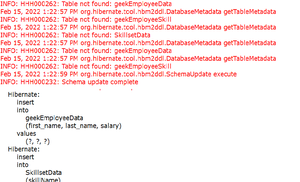Parallelly Checking the table values: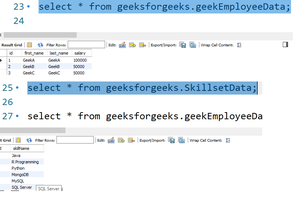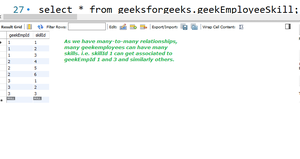geekemployeeskill data

Let us list the records by using hibernate criteria query.

By using “Set”, data is stored, while retrieving also needs to use “Set” to achieve it. As we have used hibernate mapping to have <set>, it is easier to get the skillsets. Hibernate helps to achieve it easily. We no need to write complex inner join queries to achieve that. As we have set “show_sql” as true, in the console we can see the generated query. It is shown in the screenshot below.

Example:

## Java

 `// Java Program to List the Records by``// Using Hibernate Criteria Query` `// Method``public` `void` `listGeekEmployeeData()``{` `    ``Session session = factory.openSession();``    ``Transaction tx = ``null``;` `    ``// Try block to check for exceptions``    ``try` `{``        ``tx = session.beginTransaction();` `        ``Criteria geekEmployeeCriteria``            ``= session.createCriteria(``                ``GeekEmployeeData.``class``);``        ``List geekEmployeeList = geekEmployeeCriteria.list();` `        ``for` `(Iterator iterator``             ``= geekEmployeeList.iterator();``             ``iterator.hasNext();) {` `            ``GeekEmployeeData employeeData``                ``= (GeekEmployeeData)iterator.next();``            ``System.out.print(``"First Name: "``                             ``+ employeeData.getFirstName());``            ``System.out.print(``"  Last Name: "``                             ``+ employeeData.getLastName());``            ``System.out.println(``"  Salary: "``                               ``+ employeeData.getSalary());` `            ``// As we have used Set to store data, during``            ``// display``            ``// also we need to use the set` `            ``// Iterate and get the skills one by one.``            ``// An employee may have more than 1 skill``            ``Set skillSets = employeeData.getSkillSets();` `            ``for` `(Iterator it = skillSets.iterator();``                 ``it.hasNext();) {``                ``SkillsetData skillName``                    ``= (SkillsetData)it.next();``                ``System.out.println(``                    ``"SkillName: "``                    ``+ skillName.getSkillName());``            ``}``        ``}` `        ``tx.commit();``    ``}` `    ``// Catch block to handle exceptions``    ``catch` `(HibernateException e) {` `        ``if` `(tx != ``null``)``            ``tx.rollback();``        ``e.printStackTrace();``    ``}` `    ``// finally block which will execute for sure``    ``finally` `{` `        ``// Closing all sessions``        ``// using close() method``        ``session.close();``    ``}``}`

## Java

 `/* List down all the employees by using Hibernate criteria */``geekEmployeeObject.listGeekEmployeeData();`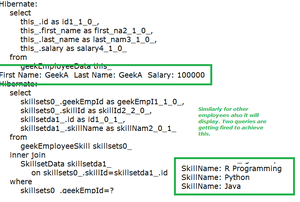While listing out employee data, we can even filter out data as well for data which is having many-to-many relationships. Via the below code, we can get the details of geekemployee who is earning more than 50000 and their firstname that should start with Geek, and the rest can be anything. “Restrictions” are getting used to achieve that. As we are adding 2 conditions, it is set with “And” in between. Here also by using “Set” we can able to list out the skillsets of the filtered employee(s) alone.

Example

## Java

 `// Java Program to illustrate Listing Employee Data` `// Method``// Listing gfgEmployee Data``// Whose firstname like certain name``// and salary > certain value` `// We can combine expressions using 'And','Or'``public` `void` `listGeekEmployeesByNameAndSalaryCriteria()``{``    ``Session session = factory.openSession();``    ``Transaction tx = ``null``;` `    ``// Try block to check for exceptions``    ``try` `{``        ``tx = session.beginTransaction();` `        ``// This will simply return every object that``        ``// corresponds to the GeekEmployee class.` `        ``Criteria geekEmployeeCriteria``            ``= session.createCriteria(``                ``GeekEmployeeData.``class``);` `        ``// Here 2 expectations are there one with salary and``        ``// second one is name.``        ``// Both are expected to be present. Let us see how``        ``// to do that``        ``Criterion salaryExpectation``            ``= Restrictions.gt(``"salary"``, ``50000``);` `        ``Criterion nameExpectation``            ``= Restrictions.ilike(``"firstName"``, ``"Geek%"``);` `        ``LogicalExpression logicalAndExpression``            ``= Restrictions.and(salaryExpectation,``                               ``nameExpectation);``        ``geekEmployeeCriteria.add(logicalAndExpression);` `        ``// As a list we can collect them and can iterate``        ``List geekEmployeeList = geekEmployeeCriteria.list();` `        ``for` `(Iterator iterator``             ``= geekEmployeeList.iterator();``             ``iterator.hasNext();) {``            ``GeekEmployeeData employeeData``                ``= (GeekEmployeeData)iterator.next();` `            ``// Print statements``            ``System.out.print(``"First Name: "``                             ``+ employeeData.getFirstName());``            ``System.out.print(``"  Last Name: "``                             ``+ employeeData.getLastName());``            ``System.out.println(``"  Salary: "``                               ``+ employeeData.getSalary());` `            ``Set skillSets = employeeData.getSkillSets();` `            ``for` `(Iterator it = skillSets.iterator();``                 ``it.hasNext();) {``                ``SkillsetData skillName``                    ``= (SkillsetData)it.next();` `                ``// Print statement``                ``System.out.println(``                    ``"SkillName: "``                    ``+ skillName.getSkillName());``            ``}``        ``}``        ``tx.commit();``    ``}` `    ``// Catch block to handle exceptions``    ``catch` `(HibernateException e) {``        ``if` `(tx != ``null``)``            ``tx.rollback();` `        ``// Display exception along with line number``        ``// using printStacktrace() method``        ``e.printStackTrace();``    ``}` `    ``// finally block which will execute for sure``    ``finally` `{` `        ``// Closing the connections``        ``// to avoid memory leakage``        ``session.close();``    ``}``}`

## Java

 `// Let us add filter data by using Restrictions``geekEmployeeObject.listGeekEmployeesByNameAndSalaryCriteria();`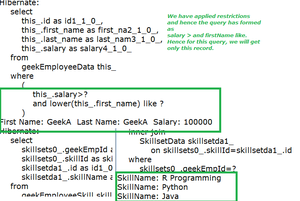Video explanation:

Conclusion: Writing complex queries, multiple inner joins make the retrieval of data in RDBMS. The same can be achieved in hibernate in an easier and user-friendly manner. Whenever there is a need of connecting more than 1 table, relationships need to be formed. Hibernate make the relationship easier way.

My Personal Notes arrow_drop_up